﻿ Verification of Rectangular RC Cross-Section | NZS 3101-2006 | GEO5 | Online nápověda

Odkaz jsme Vám odeslali na email.

Nepodařilo se nám odeslat odkaz na váš email. Zkontrolujte, prosím, Váš email.

# Online nápověda

Stromeček
Nastavení
Produkt:
Program:
Jazyk:

## Verification of Rectangular RC Cross-Section

The cross-section is rectangular, unilaterally reinforced and loaded by the bending moment and normal compression force. The program verifies a reinforced concrete section using the method of limit deformation. The maximum allowable strain of concrete in compression is 0.003. Compression reinforcement is not taken into account.

The computed degree of reinforcement is checked using the following expressions: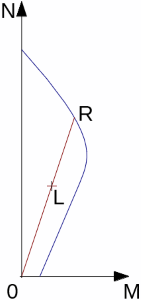Interaction diagram N-M

Usage ratio of concrete cross-section subject to the combination of bending moment and normal force is determined as |0L| / |0R|. Where L is load and R is strength with prescribed eccentricity.

## Bending without normal force

The cross-section is rectangular, reinforced on one side and loaded by the bending moment M*.

The ultimate moment is provided by: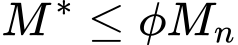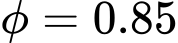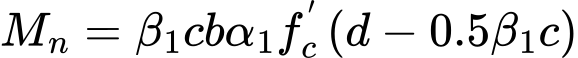for fc' < 55 MPa is α1 = 0.85

for concrete with greater strength is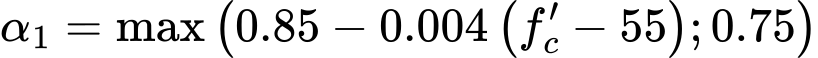for fc' < 30 MPa is β1 = 0.85

for concrete with greater strength is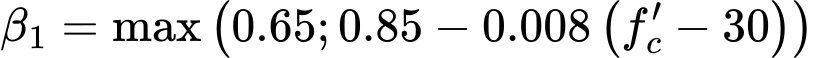The program further checks whether the location of neutral axis c is less than the limit location of neutral axis 0.75*cb given by:## Shear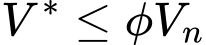where: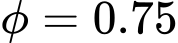First, the program computes the ultimate shear strength of concrete Vc.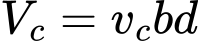for cross-sections with height smaller than 200 mm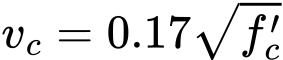vc is computed acording to following formulas for cross-sections with height greater than 400 mm, intermediate values are obtained using linear interpolation method.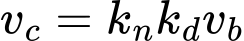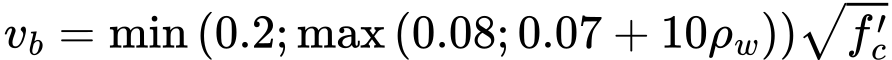where ρw is degree of reinforcement and fc' is limited to value 50 MPa.

If the ultimate shear strength of concrete is exceeded, the ultimate shear strength Vmax is checked.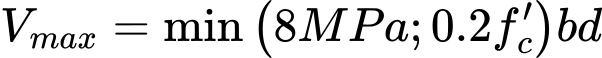Next, the necessary reinforcement area is given by: